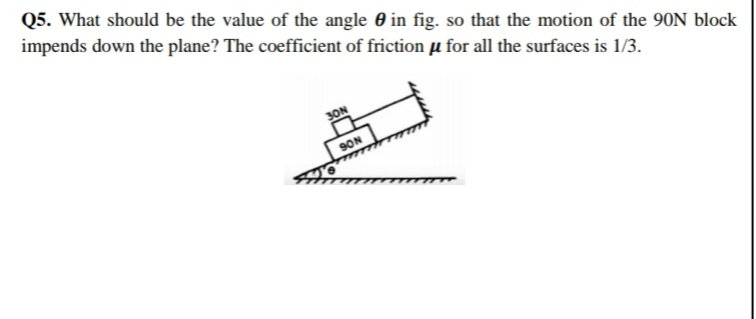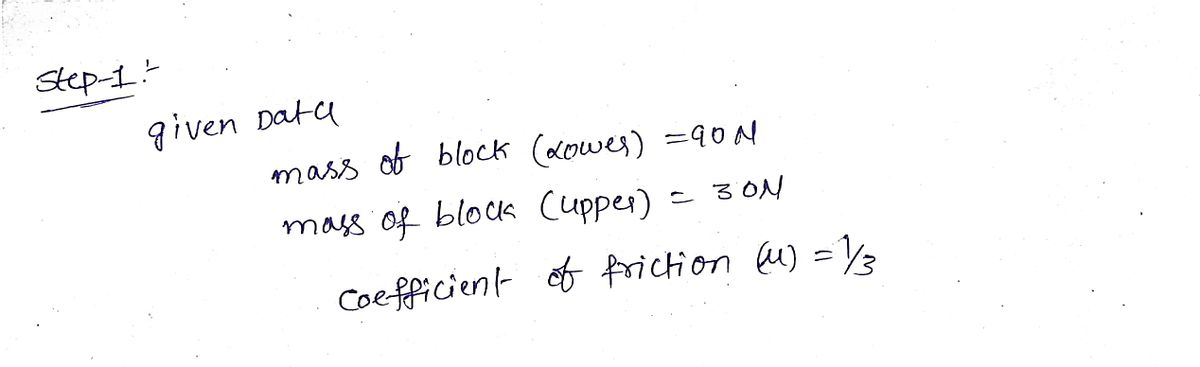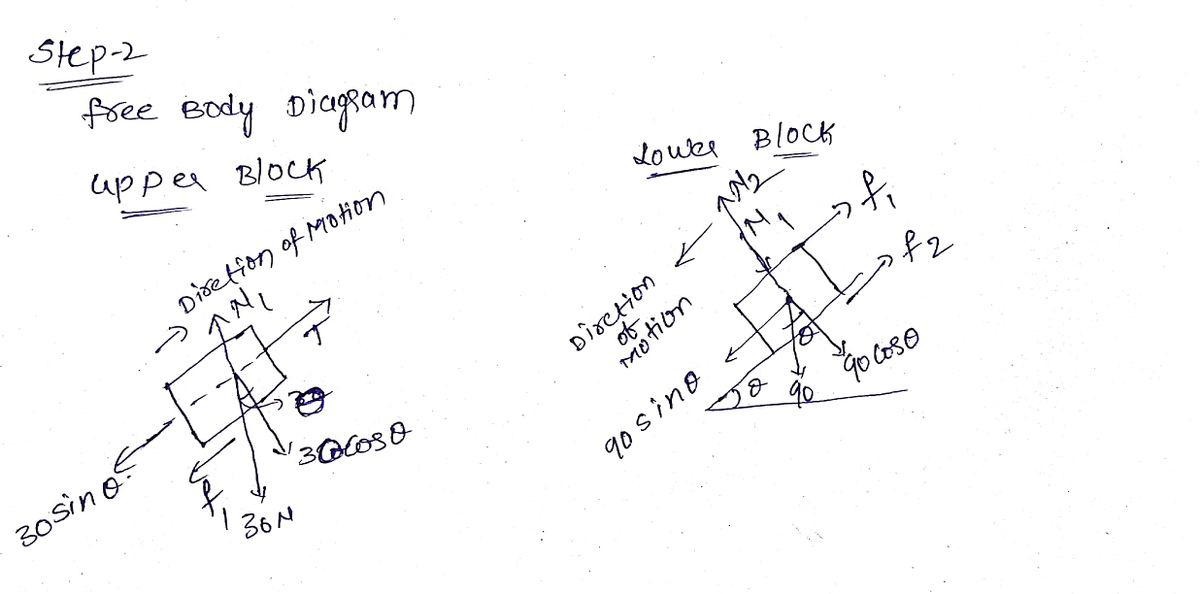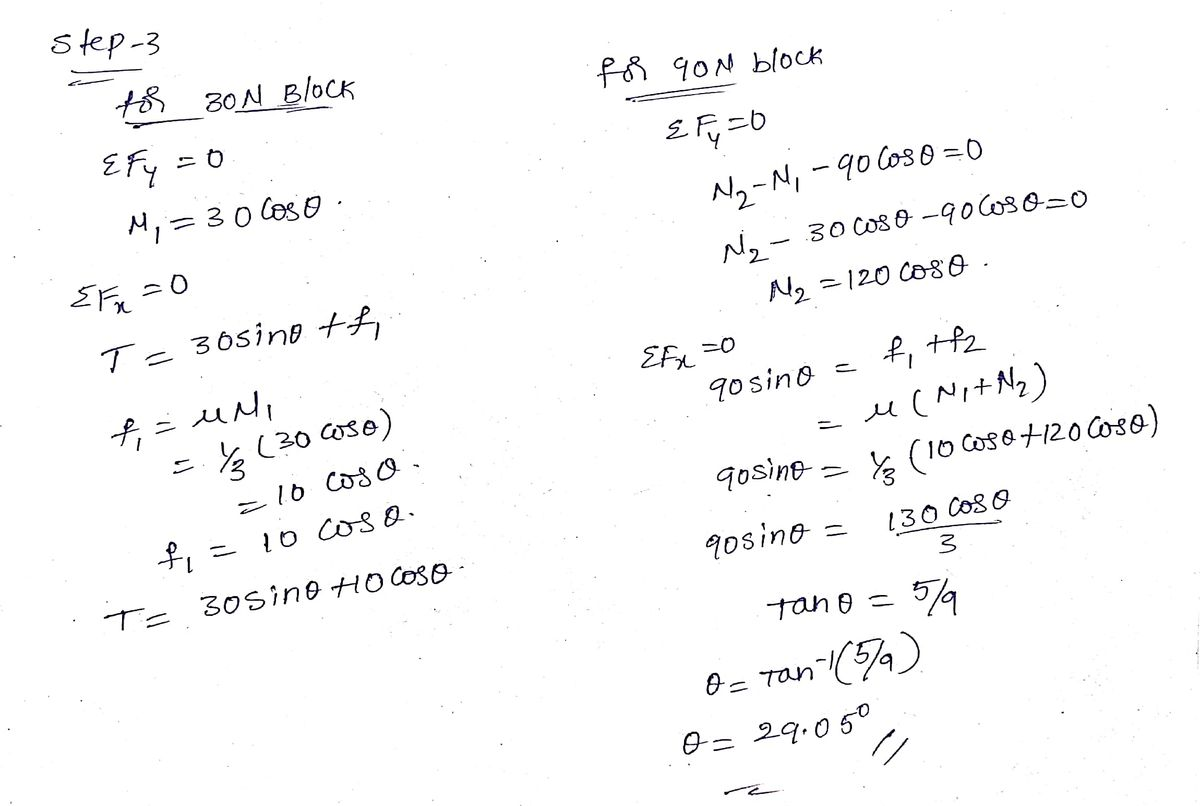# What should be the value of the angle 0 in fig. so that the motion of the 90N block impends down the plane? The coefficient of friction µ for all the surfaces is 1/3.

Question-AnswerCategory: Engineering MechanicsWhat should be the value of the angle 0 in fig. so that the motion of the 90N block impends down the plane? The coefficient of friction µ for all the surfaces is 1/3.

What should be the value of the angle 0 in fig. so that the motion of the 90N block impends down the plane? The coefficient of friction µ for all the surfaces is 1/3.Step: 1Step: 2Step: 3